Thinking

# Thales: Ancient Greeks built the cosmos with right triangles

The ancient Greeks were obsessed with geometry, which may have formed the basis of their philosophical cosmology.

Key Takeaways
• Every triangle inscribed inside a circle on its diameter is a right triangle.
• Upon this discovery, Thales is said to have performed a great ritual sacrifice.
• Might Thales have believed that the entire cosmos was constructed of right triangles?

Thales is credited by the late commentator Proclus, on the authority of Aristotle’s student Eudemus, with “discovering” geometrical propositions, some of them more generally and others more practically. Consider some of the diagrams expressing practical examples of right-angled triangles.

From left to right, we have Thales’ measurement of (i) the height of a pyramid when its shadow is equal to its height; (ii) the height of a pyramid when its shadow is unequal but proportional to its height; (iii) the distance to a ship at sea from the shoreline; and (iv) the distance to a ship at sea from a tower. Note that, when rotated, they are all the same diagram!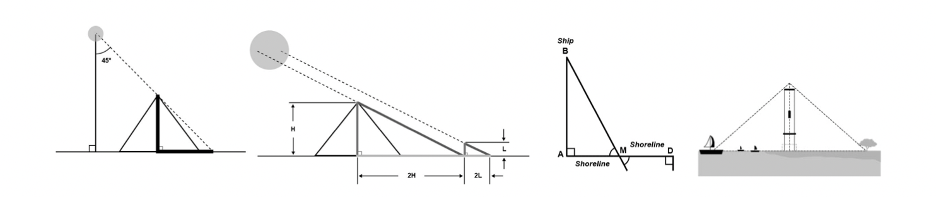The more general propositions also seem to be relevant to practical geometry: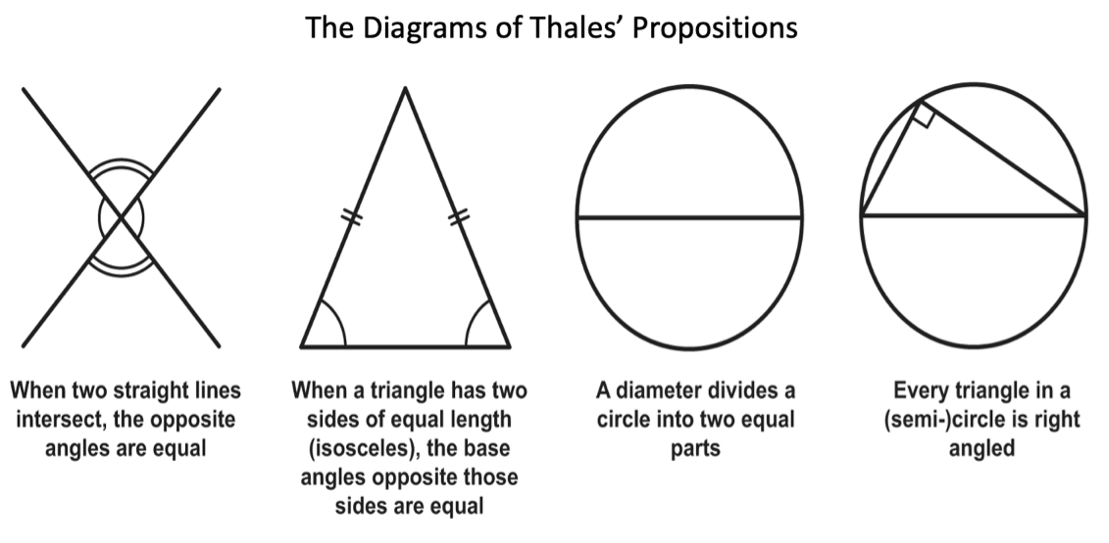We have a report about a special accomplishment of Thales. Originating with Diogenes Laertius of the 3rd century BCE on the authority of the mathematician Pamphila, it says that Thales made a splendid ritual sacrifice upon inscribing a right triangle in a circle. Obviously, he thought this was a pretty big deal. More on that a bit later.

The first thing Thales had to know is that the angles of every triangle sum to two right angles. (The angles inside every triangle sum to 180°. Two right angles, each of which is 90°, also sum to 180°.) We have an ancient report that credits Thales’ generation of geometers with having grasped this fact in all species of triangles — equilateral, isosceles, and scalene. How might Thales and his geometers have done it? Consider the following diagrams: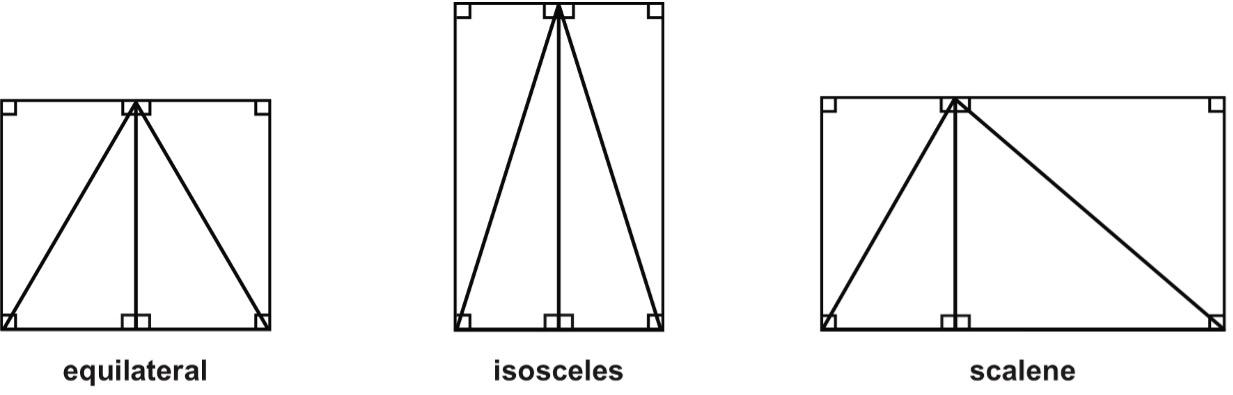By dropping a perpendicular from a vertex to the opposite side in each species of triangle, and then completing the two rectangles formed, one can see immediately that each rectangle (containing four right angles) is halved by the diagonal created by each side of the triangle. Therefore, each half-triangle contains two right angles. And if the two right angles at the base are removed, leaving the three angles of one large triangle, the angles sum to two right angles.

Now, consider how Thales may have proved that every triangle inscribed inside a circle on its diameter must be a right triangle. To show this, he relied on the isosceles triangle proposition and proved that the angle at A [α + β] is right-angled.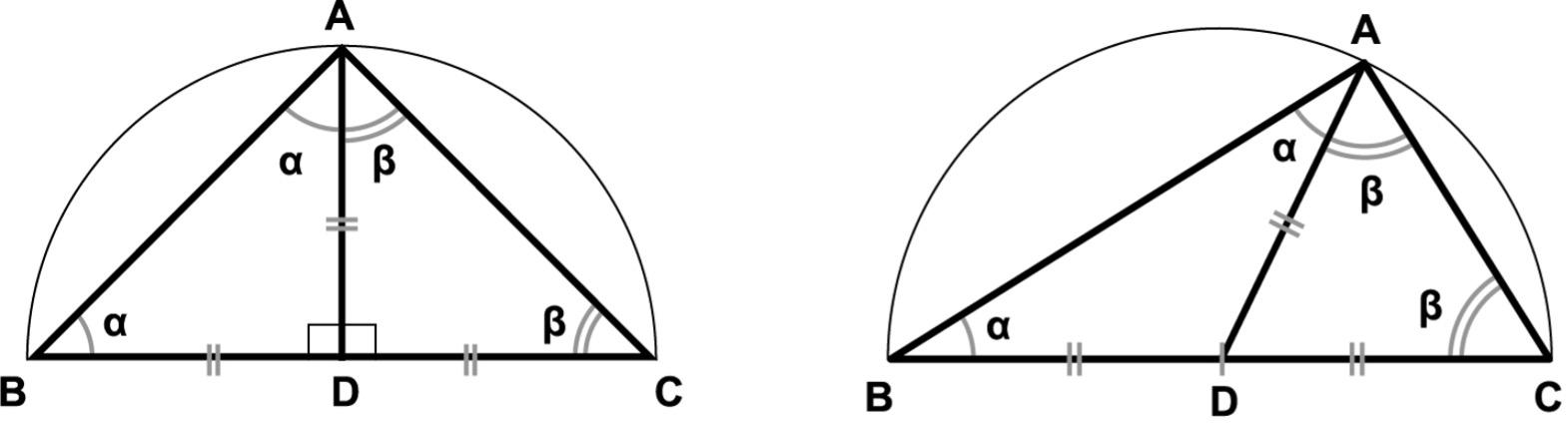Perhaps he did it this way: Based upon the isosceles triangle proposition, Thales knows that segments BD and AD (left diagram) are equal in length because they are both radii of the circle BAC. Thus, their opposite angles — α and α — must be equal. Since every triangle is 180° (that is, contains the equivalent of two right angles) and the angle BDA at the base is a right angle, α + α must also equal one right angle. By itself, α is half of a right angle.

Next, CD and AD are both equal in length since they, too, are both radii of the circle BAC, and so the angles opposite each must also be equal — that is, β equals β. If we acknowledge that the angle at the base ADC is a right angle, and there is the equivalent of two right angles in every triangle, then β + β must equal one right angle. By itself, β is half of a right angle.

Finally, the angle at A is divided into two equal parts, α and β. Because each is half of a right angle, together (α + β) they equal one right angle.

That explains the right angle for an isosceles triangle inscribed inside of a circle. But what about all the varieties of the scalene? More or less, it’s the same argument.

Consider triangle ABC (right diagram). It is composed of two triangles ABD and ACD. In ABD, AD must be equal to BD because both are radii of the circle BAC, and so the angles opposite those sides also must be equal. The same argument applies for triangle ADC. Thus, the three angles of triangle ABC are α + β + (α + β). Since we already know that the angles of every triangle sum to 180° (that is, the equivalent of two right angles), then α + β + (α + β) equals two right angles. Thus, α + β must equal one right angle.

Perhaps these lines of proof persuaded Thales and his companions that every triangle inscribed in a circle on its diameter is right. But why the great ritual sacrifice?

The ancient traditions do not give us more insight, and we are left only to speculate. Aristotle claims that Thales posited an underlying unity, water, that alters without changing. Although things look different, water is the substrate of all appearances. Water is merely altered without changing substantially. Had Thales been looking into geometry to try to discover the underlying structure of water, perhaps he followed a similar line of thought as Plato did when he identified the four elements (fire, air, water, and earth) with geometric shapes.

Thales may have identified the right triangle as the fundamental structure of water. Moreover, he now had a way to produce an unlimited number of them for further investigation simply by making a circle, drawing its diameter, and inscribing a triangle inside it.

But there is perhaps another reason for his splendid sacrifice, seen in this metaphysical light. I can imagine one of his compatriots objecting, upon hearing Thales’ idea that water was the underlying nature or unity of all things and that the right triangle was its structure. The objection may have gone like this: right triangles may form the basis of every rectilinear figure, but they certainly don’t form the basis of the circle. The circle is not constructed out of right triangles, is it? Thus, the right triangle is not the fundamental figure of all appearances.

Thales’ reply must have been as astonishing to his compatriots as it is to many of us today. Indeed, the circle too is built out of right triangles! If we plot on the circle’s diameter all the possible triangles inscribable inside a circle — starting from one end of the diameter, touching the circle, and then finishing at the other end of the diameter — we produce what modern mathematicians call a “geometrical loci.” The circle itself is constructed out of right triangles!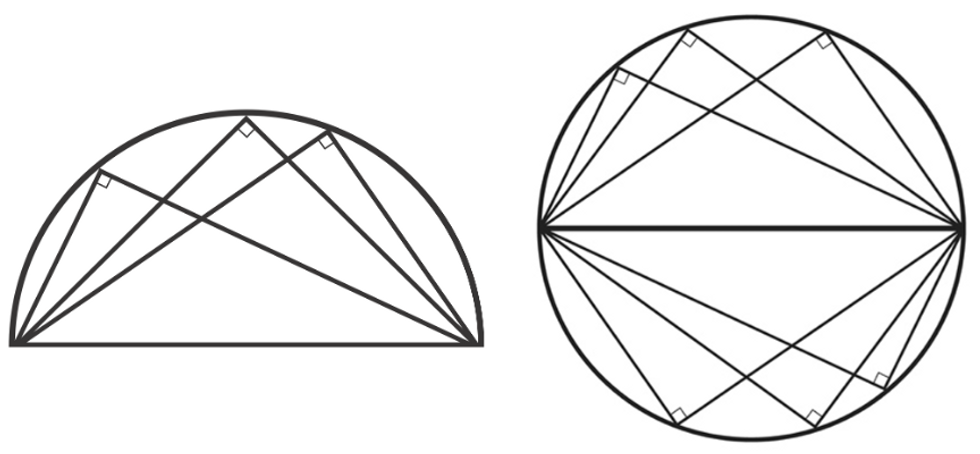Prof. Robert Hahn has broad interests in the history of ancient and modern astronomy and physics, ancient technologies, the contributions of ancient Egypt and monumental architecture to early Greek philosophy and cosmology, and ancient mathematics and geometry of Egypt and Greece. Every year, he gives “Ancient Legacies” traveling seminars to Greece, Turkey, and Egypt. His latest book is The Metaphysics of the Pythagorean Theorem.

Related

Up Next